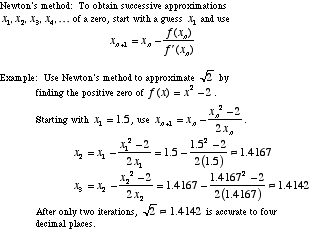index: click on a letter A B C D E F G H I J K L M N O P Q R S T U V W X Y Z A to Z index index: subject areas numbers & symbols sets, logic, proofs geometry algebra trigonometry advanced algebra & pre-calculus calculus advanced topics probability & statistics real world applications multimedia entrieswww.mathwords.com about mathwords website feedback

 Newton's Method An iterative process using derivatives that can often (but not always) be used to find zeros of a differentiable function. The basic idea is to start with an approximate guess for the zero, then use the formula below to turn that guess into a better approximation. This process is repeated until, after only a few steps, the approximation is extremely close to the actual value of the zero. Note: In some circumstances, Newton's method backfires and gives successively worse and worse approximations.